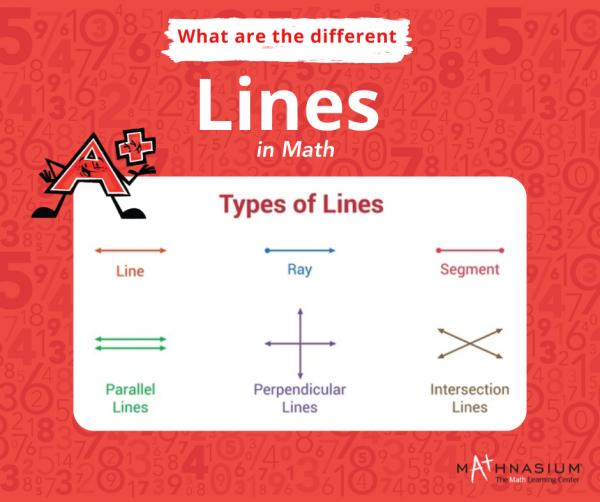* indicates a required field• phone number format invalid• email format invalidProblems detected, please review the form.
Privacy - Terms

If Hillsboro School District closes due to weather alerts, we will close also; ONLINE and IN-CENTER instruction now available!

# News from Mathnasium of Hillsboro

### Math without pen and paper

Jan 11, 2022Imagine someone asks you to calculate 3845 x 25 or 20% of 780. What would you do?

Sit with a paper and pen to work through the long multiplication? Alternatively, you can apply these newly acquired math calculation tricks.

Being able to do quick mental math can greatly help you in life. For example, if you are out buying clothes and find a shirt 15% off, are you going to take out a pen and paper and start calculating? If so, I hope you have a light backpack!.

Sometimes calculations are done quickly not in their heads, but on their fingertips. Mental math is a great exercise for the brain and can boost a student's math confidence. It also helps solve higher-level math problems at an accelerated speed. These skills are not innate, so here are some simple methods and techniques to improve your mental math.

Avoid using calculating devices.

If you want your child to hone their mental math skills, they have to stop leaning on calculators. Calculators take the necessity of mental calculation out of the equation! Start your child off easy with simple mental math queries. As they progress with addition, subtraction, multiplication and division, move to more challenging topics like percentages. Moreover, when a child encounters real life math problems, encourage them and set time to solve it. Follow these tips and it will help improve their calculation speed.

Apply in real-life

Math is a practical subject. The more we establish links between learning and real-life examples, the better can students apply their knowledge and skills and understand that the learning is valuable and relevant. How many times do you hear kids say “when are we going to use this in real life?” when they receive an assignment they don’t want to do? Showing them examples in real life is key to engaging them in their studies.

Regular practice

Practice makes a man perfect. Mathematics is a practical subject. We all start simple, and take on more challenging problems as they arise in school or in the world around us. We only get better if we regularly practice these calculations in our brains, using our mental abilities. So take a little time every day to go over the more practical side of math with your child.

Practice tricks and strategies

Test out different ways to perform mental math.  Students can try to do the calculations on their fingers, and eventually, they will develop the habit of calculating in their minds. Mental calculations involve building techniques regarding specific problems rather than memorizing answers. You can use various tools and games to develop these mental strategies. Take some time and make flashcards, watch online videos, and do math puzzles.These can help your child reinforce the new techniques they’ve learned.

Number talks

Yes, number talks are a perfect way to illustrate that math is creative. It is also a great way to begin math lessons. To begin with, pose an abstract math problem.

For example: Take 18 x 5 and ask students to try and solve it in their minds. Naturally, in a class of 20+ students, we are likely to find they answered correctly -- but differently.

Different Ways to Solve 18 x 5

 20 x 5 = 100 2 x 5 = 10 100 - 10 = 90 10 x 5 = 50 8 x 5 = 40 50 + 40 = 90 9 x 5 = 45 45 x 2 = 90 18 x 2 = 36 2 x 36 = 72 18 + 72 = 90

Mental math is all about training the mind to look beyond the obvious and calculate the solution to a problem using easy methods. Regular practice of mental math problems helps a child improve number sense and understand the math concepts better.

At Mathnasium, we encourage our students to explore math and figure out what makes the most sense to them. We empower them to take math and make it their own.